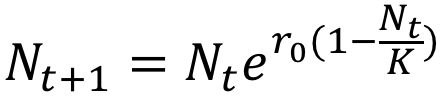# Equation of the Month

A blog run by the

Theoretical Population Ecology and Evolution Group,

Biology Dept.,

Lund University

The purpose of this blog is to emphasize the role of theory for our understanding of natural, biological systems. We do so by highlighting specific pieces of theory, usually expressed as mathematical 'equations', and describing their origin, interpretation and relevance.

## Friday, February 7, 2014

### The Ricker (logistic) modelwhere r0 is the maximum per capita growth rate and K is the carrying capacity (equilibrium population density). The Ricker equation models the change in population density (size) from one point in time, t, to a future point in time, t+1.

What does it mean?
The Ricker model (or Ricker logistic equation) models the discrete time step (discrete generation) dynamics of a population of size N. Similar to the discrete logistic equation, K is the population size at which the growth rate of the population (Nt+1Nt) is zero. This does however not mean that birth and deaths are absent, rather that births and deaths are equal and cancel each other out. Per capita growth (Nt+1 / Nt) is a decreasing function of Nt and is maximized (and equal to exp(r0)) when Nt = 0.

Where does it come from?
The model was first introduced in Ricker (1954) where it was used to model stock dynamics and recruitment in fisheries. The model is similar to (in terms of formulization and dynamical behavior) and inspired by the logistic growth equation, however, it is formulized in a way such that Nt+1 cannot become negative, even if Nt >> K. Consequently, it is somewhat more realistic and "safer" to use.

Importance
As for the logistic equation the Ricker model is used in theoretical ecology to model all types of population dynamics and recruitment in general, although if first was introduced to model fish populations.

Mikael Pontarp

Literature
• Ricker, W.E. 1952. Stock and recruitment. J. Fish. Res. Board Can. 11:559-623
• Case, T.J. 2000. An illustrated guide to theoretical ecology. Oxford UP
• Mangel, M. 2006. The theoretical biologists toolbox. Cambridge UP
• Brännström, Å. and Sumpter, D.J. 2005. The role of competition and clustering in population dynamics. Proc. Biol. Sci. 272(1576): 2065–72
• Geritz, S.A. and Kisdi, E. 2004. On the mechanistic underpinning of discrete-time population models with complex dynamics. J. Theor. Biol. 228(2):261–9